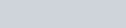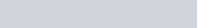# Three formulas or rules to explain shaft deflection

THREE FORMULAS OR RULES TO EXPLAIN SHAFT DEFLECTION T013

In my seminars I talk about the three magic formulas or rules you need to know if you want to understand how centrifugal pumps function. Here are the formulas I show:

• Velocity plus head must remain a constant. As the velocity of a liquid increases, the pressure (ninety degrees to the flow) will decrease and as the velocity of a liquid decreases, the pressure (ninety degrees to the flow) will increase.
• Velocity times area must remain a constant if liquid is to flow.
• Pressure acting on an area creates a force.

Let’s will look at each of these formulas in detail:

Formula #1. Velocity + head = a constant.

This formula explains how airplanes fly. It all started when the Wright brothers discovered the correct wing shape for an aircraft.

Please take a look at the following diagram. You will note that the air is flowing under the wing at some velocity. The air going over the top of the wing has a longer path to travel so its velocity must increase if it is to join with the air coming underneath the wing.The air underneath the wing is at atmospheric pressure, but since the velocity is greater on the top of the wing the pressure falls to some value below atmospheric pressure. This causes atmospheric pressure to push on the bottom of the wing, lifting the wing, the airplane, and all the people inside, up into the air. It will continue to do so as long as the wing is moving forward and the configuration of the wing does not change.

Gravity offsets this lifting force and the aircraft flies between these two forces. This same principle explains how an automobile carburetor works, why the shower curtain comes into the bath tub when you take a shower, and how a sailboat can sail faster than the wind.

Let’s skip to formula #3 to learn why the wing lifted into the air.

Formula #3: pressure x area = force.

 PRESSURE x AREA = FORCEPressure is measure in pounds per square inch (kilograms per square centimeter)Area is measured in square inches (square centimeters)The units for force then become pounds (kilograms)

It is important for us to know the forces being generated because force over distance in a given time period is a measure of work, energy expended, or heat depending upon which units we use.

Formula #2: velocity x area = a constant

This formula explains the action of a venturi and explains what happens when the traveling liquid encounters different diameter passages in the pump and pumping system.As the area inside a venturi goes down the velocity of the fluid goes up. This increase in velocity causes the pressure to decrease (formula #1) allowing atmospheric pressure to push a fluid into the venturi.

We use the venturi principle to push paint to a spray gun, remove air from a condenser, add chemical to a boiler etc. It is the same principle we use to get fuel to the carburetor of older automobiles.

Now we will look at the cross section of a centrifugal pump and these three formulas will explain why mechanical seals have so much trouble with shaft deflection.This illustration describes a volute pump because the impeller is not in the center of the casing. You will note that there is less clearance between the impeller and the cut-water than there is between the impeller and the rest of the casing.

You will also note that this volute area is increasing as you move from the cutwater, around the casing to the discharge nozzle.

Circular pumps have an equal area around the impeller. They are used to pump greater quantities of liquid without having to create a high head. The volute design is the most popular design because it does produce a head.

When we removed the packing from a centrifugal pump we lost a big part of the shaft support system. It therefore becomes very important that we keep the forces equal around the impeller to prevent shaft displacement. If the force increases on one side of the impeller it will deflect the attached shaft and interfere with the performance of the mechanical seal.

Since the impeller is symmetrical in shape (the area is the same all around the impeller) It is important that we do not let the pressure vary around the impeller or the resultant forces will not be equal (formula #3). To keep the pressure equal around the impeller, you have to keep the velocity of the liquid constant around the impeller (formula #1).

The pump could be operating at one of three points on the pump curve:

• On the right hand side of the curve where you will get a high capacity and a low head
• On the left hand side of the curve where you will get high head and low capacity
• At the best efficiency point (BEP) where the head and capacity are exactly right for that pump.

Assuming you are on the right hand side of the curve (high capacity side) the liquid velocity will increase as it approaches the discharge because there is not enough head on the system. As the liquid velocity increases the pressure will decrease (rule #1) and the shaft will deflect radially at 60° from the cutwater because pressure times area will create a force.

If you are on the left-hand side of the curve (high head) the liquid will not be able to flow out the discharge so a portion of it will recirculate by the cutwater. As the velocity of the liquid increases (rule #2) the pressure will decrease (rule #1) and the shaft will deflect radially at 240° from the cutwater because pressure times area will create a force.Between these two extremes there is a point where there will be no radial shaft deflection caused by the wrong capacity. This is called the best efficiency point (BEP) of the pump. Look at the diagram again and note those deflections:

In another section of this CD I talk about methods of stabilizing the shaft for these “off design” operations, but the fact remains that shaft deflection continues to be a major source of mechanical seal problems and will continue to be so until the pump manufacturer accepts the responsibility of building a sensible pump.

Your solutions to this deflection problem are limited because changing the shaft material will not help. You can:

• Decrease the length of the shaft.
• Increase the diameter of the shaft.
• Convert to a double volute design.
• Support the shaft in the stuffing box with some type of support bushing.
• Put in the correct size pump and operate at the best efficiency point (BEP)
• In some limited cases a variable speed driver would help.

If you would like to calculate the actual forces involved, please see: Shaft Bending

### Posted

• On February 17, 2018